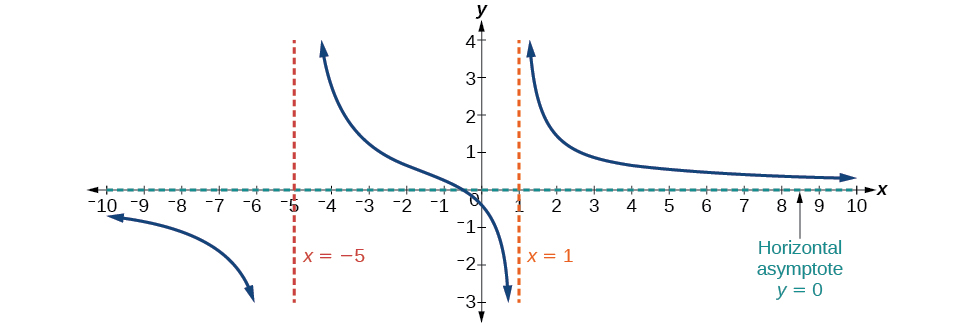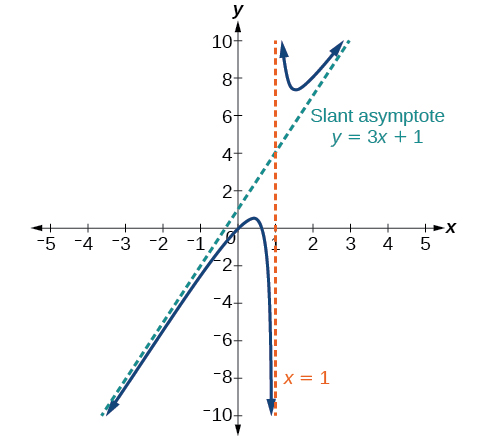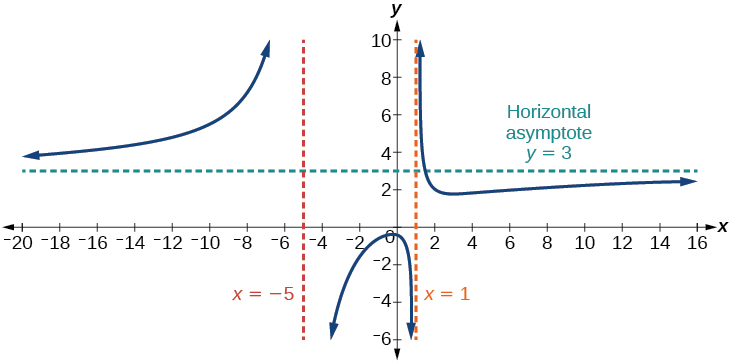# 5.6 Rational functions  (Page 5/16)

 Page 5 / 16

Find the vertical asymptotes and removable discontinuities of the graph of $\text{\hspace{0.17em}}f\left(x\right)=\frac{{x}^{2}-25}{{x}^{3}-6{x}^{2}+5x}.$

Removable discontinuity at $\text{\hspace{0.17em}}x=5.\text{\hspace{0.17em}}$ Vertical asymptotes:

## Identifying horizontal asymptotes of rational functions

While vertical asymptotes describe the behavior of a graph as the output gets very large or very small, horizontal asymptotes help describe the behavior of a graph as the input gets very large or very small. Recall that a polynomial’s end behavior will mirror that of the leading term. Likewise, a rational function’s end behavior will mirror that of the ratio of the function that is the ratio of the leading terms.

There are three distinct outcomes when checking for horizontal asymptotes:

Case 1: If the degree of the denominator>degree of the numerator, there is a horizontal asymptote    at $\text{\hspace{0.17em}}y=0.$

In this case, the end behavior is $\text{\hspace{0.17em}}f\left(x\right)\approx \frac{4x}{{x}^{2}}=\frac{4}{x}.\text{\hspace{0.17em}}$ This tells us that, as the inputs increase or decrease without bound, this function will behave similarly to the function $\text{\hspace{0.17em}}g\left(x\right)=\frac{4}{x},\text{\hspace{0.17em}}$ and the outputs will approach zero, resulting in a horizontal asymptote at $\text{\hspace{0.17em}}y=0.\text{\hspace{0.17em}}$ See [link] . Note that this graph crosses the horizontal asymptote.Horizontal asymptote   y = 0   when   f ( x ) = p ( x ) q ( x ) ,   q ( x ) ≠ 0   where degree of   p < degree of  q .

Case 2: If the degree of the denominator<degree of the numerator by one, we get a slant asymptote.

In this case, the end behavior is $\text{\hspace{0.17em}}f\left(x\right)\approx \frac{3{x}^{2}}{x}=3x.\text{\hspace{0.17em}}$ This tells us that as the inputs increase or decrease without bound, this function will behave similarly to the function $\text{\hspace{0.17em}}g\left(x\right)=3x.\text{\hspace{0.17em}}$ As the inputs grow large, the outputs will grow and not level off, so this graph has no horizontal asymptote. However, the graph of $\text{\hspace{0.17em}}g\left(x\right)=3x\text{\hspace{0.17em}}$ looks like a diagonal line, and since $\text{\hspace{0.17em}}f\text{\hspace{0.17em}}$ will behave similarly to $\text{\hspace{0.17em}}g,\text{\hspace{0.17em}}$ it will approach a line close to $\text{\hspace{0.17em}}y=3x.\text{\hspace{0.17em}}$ This line is a slant asymptote.

To find the equation of the slant asymptote, divide $\text{\hspace{0.17em}}\frac{3{x}^{2}-2x+1}{x-1}.\text{\hspace{0.17em}}$ The quotient is $\text{\hspace{0.17em}}3x+1,\text{\hspace{0.17em}}$ and the remainder is 2. The slant asymptote is the graph of the line $\text{\hspace{0.17em}}g\left(x\right)=3x+1.\text{\hspace{0.17em}}$ See [link] .Slant asymptote when   f ( x ) = p ( x ) q ( x ) ,   q ( x ) ≠ 0   where degree of   p > degree of  q   by   1 .

Case 3: If the degree of the denominator = degree of the numerator, there is a horizontal asymptote at $\text{\hspace{0.17em}}y=\frac{{a}_{n}}{{b}_{n}},\text{\hspace{0.17em}}$ where $\text{\hspace{0.17em}}{a}_{n}\text{\hspace{0.17em}}$ and $\text{\hspace{0.17em}}{b}_{n}\text{\hspace{0.17em}}$ are the leading coefficients of $\text{\hspace{0.17em}}p\left(x\right)\text{\hspace{0.17em}}$ and $\text{\hspace{0.17em}}q\left(x\right)\text{\hspace{0.17em}}$ for $\text{\hspace{0.17em}}f\left(x\right)=\frac{p\left(x\right)}{q\left(x\right)},q\left(x\right)\ne 0.$

In this case, the end behavior is $\text{\hspace{0.17em}}f\left(x\right)\approx \frac{3{x}^{2}}{{x}^{2}}=3.\text{\hspace{0.17em}}$ This tells us that as the inputs grow large, this function will behave like the function $\text{\hspace{0.17em}}g\left(x\right)=3,\text{\hspace{0.17em}}$ which is a horizontal line. As $\text{\hspace{0.17em}}x\to ±\infty ,f\left(x\right)\to 3,\text{\hspace{0.17em}}$ resulting in a horizontal asymptote at $\text{\hspace{0.17em}}y=3.\text{\hspace{0.17em}}$ See [link] . Note that this graph crosses the horizontal asymptote.Horizontal asymptote when   f ( x ) = p ( x ) q ( x ) ,   q ( x ) ≠ 0   where degree of  p = degree of  q .

Notice that, while the graph of a rational function will never cross a vertical asymptote    , the graph may or may not cross a horizontal or slant asymptote. Also, although the graph of a rational function may have many vertical asymptotes, the graph will have at most one horizontal (or slant) asymptote.

root under 3-root under 2 by 5 y square
The sum of the first n terms of a certain series is 2^n-1, Show that , this series is Geometric and Find the formula of the n^th
cosA\1+sinA=secA-tanA
why two x + seven is equal to nineteen.
The numbers cannot be combined with the x
Othman
2x + 7 =19
humberto
2x +7=19. 2x=19 - 7 2x=12 x=6
Yvonne
because x is 6
SAIDI
what is the best practice that will address the issue on this topic? anyone who can help me. i'm working on my action research.
simplify each radical by removing as many factors as possible (a) √75
how is infinity bidder from undefined?
what is the value of x in 4x-2+3
give the complete question
Shanky
4x=3-2 4x=1 x=1+4 x=5 5x
Olaiya
hi can you give another equation I'd like to solve it
Daniel
what is the value of x in 4x-2+3
Olaiya
if 4x-2+3 = 0 then 4x = 2-3 4x = -1 x = -(1÷4) is the answer.
Jacob
4x-2+3 4x=-3+2 4×=-1 4×/4=-1/4
LUTHO
then x=-1/4
LUTHO
4x-2+3 4x=-3+2 4x=-1 4x÷4=-1÷4 x=-1÷4
LUTHO
A research student is working with a culture of bacteria that doubles in size every twenty minutes. The initial population count was  1350  bacteria. Rounding to five significant digits, write an exponential equation representing this situation. To the nearest whole number, what is the population size after  3  hours?
v=lbh calculate the volume if i.l=5cm, b=2cm ,h=3cm
Need help with math
Peya
can you help me on this topic of Geometry if l help you
litshani
( cosec Q _ cot Q ) whole spuare = 1_cosQ / 1+cosQ
A guy wire for a suspension bridge runs from the ground diagonally to the top of the closest pylon to make a triangle. We can use the Pythagorean Theorem to find the length of guy wire needed. The square of the distance between the wire on the ground and the pylon on the ground is 90,000 feet. The square of the height of the pylon is 160,000 feet. So, the length of the guy wire can be found by evaluating √(90000+160000). What is the length of the guy wire?
the indicated sum of a sequence is known as
how do I attempted a trig number as a starter
cos 18 ____ sin 72 evaluateByByBy OpenStaxBy JavaChamp TeamBy Stephanie RedfernBy Abby SharpBy OpenStaxBy Richley CrapoBy Jazzycazz JacksonBy Monty HartfieldBy John GabrieliBy Stephen Voron